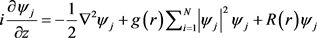﻿ 二维矢量多极孤子和涡旋孤子探究 Research on Two-Dimensional Vector Multipole Solitons and Vortex Solitons

Modern Physics
Vol. 09  No. 04 ( 2019 ), Article ID: 31366 , 5 pages
10.12677/MP.2019.94021

Research on Two-Dimensional Vector Multipole Solitons and Vortex Solitons

Xianjing Lai

Department of Physics, Basic College, Zhejiang Shuren University, Hangzhou ZhejiangReceived: Jun. 20th, 2019; accepted: Jul. 15th, 2019; published: Jul. 22nd, 2019ABSTRACT

In this paper, two-dimensional coupled nonlinear Schrödinger equations with spatial nonlinear modulation and lateral modulation are studied, and vector multipole and vortex soliton solutions are derived and analyzed. When the modulation depth is selected to be 0 and 1, the vector multipole and vortex soliton structures are obtained, respectively. The number of azimuthal lobes (the “petal" of a plurality of polarized solitons) is determined by the topological index m, and the number of layers in the multipole soliton is determined by the value of n.

Keywords:Two-Dimensional Coupled Nonlinear Schrödinger Equation, Vector Multipole Soliton, Vector Vortex Soliton1. 研究背景

2. 理论模型及形变约化(1)

${\psi }_{j}\left(r,\phi ,z\right)=A\left(r\right){\Phi }_{j}\left(\phi \right)\mathrm{exp}\left(-i\kappa z\right)$ (2)

$\frac{{r}^{2}}{A}\left\{\frac{{\partial }^{2}A}{\partial {r}^{2}}+\frac{1}{r}\frac{\partial A}{\partial r}+2\left[\kappa -R\left(r\right)\right]A-2g\left(r\right){A}^{3}\right\}={m}^{2}$ (3)

$-\frac{1}{{\varphi }_{j}}\frac{{\partial }^{2}{\varphi }_{j}}{\partial {\phi }^{2}}={m}^{2}$ (4)

${\Phi }_{j}={C}_{j}\mathrm{cos}\left(m\phi \right)+{D}_{j}\mathrm{sin}\left(m\phi \right)$ (5)

$A\left(r\right)=\rho \left(r\right)U\left[\chi \left(r\right)\right]$ 代入方程(3)，且 $U\left[\chi \left(r\right)\right]$ 满足

$-\frac{{\text{d}}^{2}U}{\text{d}{\chi }^{2}}+G\left(U\right)=\eta U$ (6)

${\rho }_{rr}+\frac{1}{r}{\rho }_{r}+\left[2\kappa -2R\left(r\right)-\frac{{m}^{2}}{{r}^{2}}\right]\rho =\frac{\eta }{{r}^{2}{\rho }^{3}}$ (7)

$\frac{G\left(U\right){\chi }_{r}^{2}}{{U}^{3}}-2g{\rho }^{2}=0$ (8)

$g\left(r\right)=G\left(U\right){r}^{-2}{\rho }^{-6}\left(r\right)/\left(2{U}^{3}\right)$$\chi \left(r\right)={\int }_{0}^{r}{\rho }^{-2}\left(s\right){s}^{-1}\text{d}s$ (9)

$\rho ={r}^{-1}\left[{c}_{1}M\left(\frac{\kappa }{2\sqrt{2\omega }},\frac{m}{2},\sqrt{2\omega }{r}^{2}\right)+{c}_{2}W\left(\frac{\kappa }{2\sqrt{2\omega }},\frac{m}{2},\sqrt{2\omega }{r}^{2}\right)\right]$ (10)

$\rho ={c}_{3}J\left(m,\sqrt{2\kappa }r\right)+{c}_{4}Y\left(m,\sqrt{2\kappa }r\right)$ (11)

$\rho =\sqrt{\frac{1}{r}\left(\alpha {\varphi }_{1}^{2}+2\beta {\varphi }_{1}{\varphi }_{2}+\gamma {\varphi }_{2}^{2}\right)}$ (12)

${\varphi }_{rr}+\left[2\kappa -2R\left(r\right)-\frac{{m}^{2}}{{r}^{2}}\right]\varphi =0$

$U\left(\chi \right)=\frac{2n\lambda }{\sqrt{-{g}_{0}}}sd\left[2n\lambda \chi \left(r\right),\frac{\sqrt{2}}{2}\right]$ (13)

3. 矢量多极孤子和涡旋孤子结构

4. 小结

Research on Two-Dimensional Vector Multipole Solitons and Vortex Solitons[J]. 现代物理, 2019, 09(04): 191-195. https://doi.org/10.12677/MP.2019.94021

1. 1. Quiroga-Teixeiro, M. and Michinel, H.J. (1997) Stable Azimuthal Stationary State in Quintic Nonlinear Optical Media. Journal of the Optical Society of America B, 14, 2004-2009.
https://doi.org/10.1364/JOSAB.14.002004

2. 2. Manakov, S.V. (1974) On the Theory of Two-Dimensional Sta-tionary Self-Focusing of Electromagnetic Waves. Soviet Physics—JETP, 38, 248-253.

3. 3. Radhakrishnan, R. and Aravinthan, K.J. (2007) A Dark-Bright Optical Soliton Solution to the Coupled Nonlinear Schrödinger Equation. Journal of Physics A: Mathematical and Theoretical, 40, Article ID: 13023.
https://doi.org/10.1088/1751-8113/40/43/011

4. 4. Sun, Z.Y., Gao, Y.T., Yu, X., Liu, W.J. and Liu, Y. (2009) Bound Vector Solitons and Soliton Complexes for the Coupled Nonlinear Schrödinger Equations. Physical Review E, 80, Article ID: 066608.
https://doi.org/10.1103/PhysRevE.80.066608

5. 5. Desyatnikov, A.S., Kivshar, Y.S. and Torner, L. (2005) Optical Vortices and Vortex Solitons. Progress in Optics, 47, 291-391.
https://doi.org/10.1016/S0079-6638(05)47006-7

6. 6. Borovkova, O.V., Kartashov, Y.V., Torner, L. and Malomed, B.A. (2011) Bright Solitons from Defocusing Nonlinearities. Physical Review E, 84, Article ID: 035602(R).
https://doi.org/10.1103/PhysRevE.84.035602

7. 7. Zhong, W.P., Belic, M.R., Assanto, G., Malomed, B.A. and Huang, T.W. (2011) Self-Trapping of Scalar and Vector Dipole Solitary Waves in Kerr Media. Physical Review A, 83, Article ID: 043833.
https://doi.org/10.1103/PhysRevA.83.043833